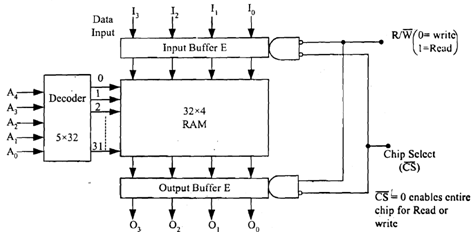## Illustrate internal organisation of ram, Computer Engineering

Assignment Help:

Q. Illustrate Internal Organisation of RAM?

The construction displayed in Figure below is made up of one JK flip-flop and 3 AND gates. The two inputs to system are one input bit and read/write signal. Input is fed in complemented form to AND gate 'a'. Read/write signal has a value of 1 if it is a read operation. So at the time of read operation AND gate 'c' has read/write input as 1. Because AND gate 'a' and 'b' have 0 read/write input and if chip is selected it implies that this cell is currently being selected then output would become equivalent to state of flip-flop. In other words data value stored in flip-flop has been read. In write operation only 'a' and 'b' gates get a read/write value of 1 and they clear or set JK flip-flop depending on data input value. If data input is 0, flip-flop will go to clear state and if data input is 1, flip-flop will go to set state. In effect input data is reflected in state of flip-flop. So we say that input data has been stored in flip-flop or binary cell.Figure: Internal Organisation of a 32 × 4 RAM

A 32 × 4 RAM means this RAM has 32 words, 5 address lines (25 = 32) and 4 bit data word size. Please note that we can signify a RAM using 2A×D where A is number of address lines and D is number of Data lines. Figure above is extension of binary cell to an integrated 32 × 4 RAM circuit where a 5 × 32 bit decoder is used. 4 bit data inputs come through an input buffer and 4-bit data output is stored in output buffer.

A chip select (C¯S¯) control signal is used as a memory enable input. When CS = 0 which is C¯S¯ = 1 it enables complete chip for read or write operation. An R/W signal can be used for read or write operation. The word which is selected will determine overall output.  Since all the above is a logic circuit of equal length which can be accessed in equal time so the word RAM.

#### Web technology, what is rowspan and colspan

what is rowspan and colspan

#### What is dynamic modelling, What is Dynamic Modelling  As you know that...

What is Dynamic Modelling  As you know that computer systems are built from the objects which respond to events. External events arrive at boundary of system; you understan

#### Fundamental difference between smart card and e-cash, What is the fundament...

What is the fundamental difference between the transactions made using Smart Card and E-cash? Smart Card and E-Cash: E-cash storable smart cards can dispense and store ca

#### What is gimp?, The GNU Image Manipulation Program, or GIMP, is a raster gra...

The GNU Image Manipulation Program, or GIMP, is a raster graphics editor application with some support for vector graphics. GIMP is used to process digital graphics & photographs.

#### What is load testing, Load testing is to test that if the application works...

Load testing is to test that if the application works well with the loads that result from large number of concurrent users, transactions and to verify whether it can handle peak u

#### Data structures, what are data structures

what are data structures

#### Electing neil, ELECTING NEIL : The response for this is, of course. In...

ELECTING NEIL : The response for this is, of course. In this case, we considered the point of the search or researching is to find an artifact  -  a word which is an anagram o

#### cellfun function, There is a built-in function function known as cellfun t...

There is a built-in function function known as cellfun that evaluates a function for each element of a cell array.  Make a cell array, then call the cellfun function, passing the h

#### Voltage tripler and voltage quadrupler.., explan volage triper and voltage ...

explan volage triper and voltage quadrupler.

#### Representation scheme in artificial intelligence, Representation scheme in ...

Representation scheme in artificial intelligence: It is not hard to see why logic has been popular representation scheme in AI: In this way, It is easy to represent knowl